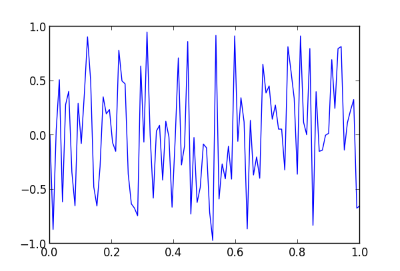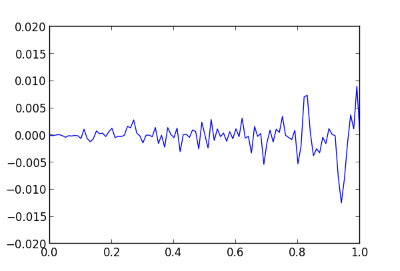Over a million developers have joined DZone.{{announcement.body}}
{{announcement.title}}

# Exact Chaos

DZone 's Guide to

# Exact Chaos

· Big Data Zone ·
Free Resource

Comment (0)

Save
{{ articles.views | formatCount}} Views

Pick a number x between 0 and 1. Then repeatedly replace x with 4x(1-x). For almost all starting values of x, the result exhibits chaos. Two people could play this game with starting values very close together, and eventually their sequences will diverge.

It’s somewhat surprising that the iterative process described above can be written down in closed form. Starting from a value x0, the value after n iterations is

sin( 2n arcsin( √ x0 ) )2.

Now suppose two people start with the same initial value. One repeatedly applies 4x(1-x) and the other uses the formula above. If both carried out their calculations exactly, both would produce the same output at every step. But what if both used a computer?

The two approaches correspond to the Python functions `f` and `g` below. Because both functions are executed in finite precision arithmetic, both have errors, but they have different errors. Suppose we want to look at the difference between the two functions as we increase `n`.

```from scipy import arcsin, sin, sqrt, linspace
from matplotlib import pyplot as plt

def f(x0, n):
x = x0
for _ in range(n):
x = 4*x*(1-x)
return x

def g(x0, n):
return sin(2.0**n * arcsin(sqrt(x0)))**2

n = 40
x = linspace(0, 1, 100)
plt.plot(x, f(x, n) - g(x, n))
plt.ylim(-1, 1)
plt.show()```

When we run the code, nothing exciting happens. The difference is a flat line.Next we increase `n` to 45 and we start to see the methods diverge.The divergence is large when `n` is 50.And the two functions are nearly completely uncorrelated when `n` is 55.Update

So which function is more accurate, `f` or `g`? As noted in the comments, the two functions have different kinds of numerical errors. The former accumulates arithmetic precision error at each iteration. The latter shifts noisy bits into significance by multiplying by 2^n. Apparently both about the same overall error, though they have different distributions of error.

I recomputed `g` using 100-digit precision with `mpmath` and used the results as the standard to evaluate the output of `f` and `g` in ordinary precision. Here’s a plot of the errors when `n` = 45, first with `f`and then with `g`.The average absolute errors of `f` and `g` are 0.0024 and 0.0015 respectively.

Topics:

Comment (0)

Save
{{ articles.views | formatCount}} Views

Published at DZone with permission of

Opinions expressed by DZone contributors are their own.